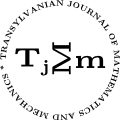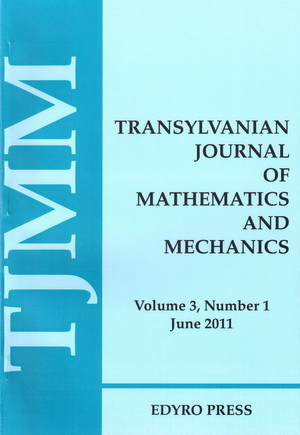ISSN: 2067-239X
ISSN(on-line): 2067-239X

Indexed in:
Mathematical Reviews
Zentralblatt MATH
EBSCO

Front cover# Volume 8 (2016), Number 2

## On the Certain Subclasses of Univalent Functions Associated With the Tschebyscheff Polynomials

### Author(s): ŞAHSENE ALTINKAYA and SIBEL YALÇIN

Abstract: In this work, considering certain subclasses of univalent functions and using the Tschebyscheff polynomials, we obtain coefficient expansions and solve the Fekete-Szegö problem for the functions that belong to these new subclasses.

## Stability in Nonlinear Neutral Volterra Integral Equations on a Time Scale

### Author(s): ABDELOUAHEB ARDJOUNI and AHCENE DJOUDI

Abstract: Let $𝕋$ be a time scale that is unbounded above and below and such that $0\in 𝕋$. Let $id-\tau :𝕋\to 𝕋$ be such that $\left(id-\tau \right)\left(𝕋\right)$ is a time scale. We use the contraction mapping theorem to obtain stability results about the zero solution of the nonlinear neutral Volterra integral equation with variable delay

where ${f}^{△}$ is the $△$-derivative on $𝕋$ and ${f}^{\stackrel{̃}{△}}$ is the $△$-derivative on $\left(id-\tau \right)\left(𝕋\right)$. The results obtained here extend some results due to Raffoul .

## A Simpliﬁed Mathematical Model of the Universe Expansion and an Estimation of Its Life Time Duration

### Author(s): MALVINA BAICA and MIRCEA CARDU

Abstract: In this paper the authors represent their argument in establishing a most simple Mathematical Model as they could, in order to represent the Universe Expansion from the moment of its apparition (Big-Bang). We start from the fact that out of the total of 13.7 billion of years elapsed from this moment (B-B) up to the present, on the last portion of this interval about $90%$ of it, the curve which represent the Universe spatial dimension variation in function of time can be equivalent, with a suﬃcient large precision, to an arc of a circle.

## New Inﬂuence Functions for Thermal Displacements and Stresses Within Half-Space

### Author(s): ION CREȚU

Abstract: In this study new inﬂuence functions for the thermal displacements and stresses caused by a unit point heat source for three-dimensional boundary value problem of thermoelasticity within half-space were obtained. These results are presented in terms of elementary functions. Using the computer program Maple 18, the graphical presentations of thermal displacements and stresses caused by a unit point heat source were constructed.

## New Integral Inequalities of the Type of Simpson’s and Hermite-Hadamard’s for Twice Differentiable Quasi-Geometrically Convex Mappings

### Author(s): MUHAMMAD MUDDASSAR and ZAFFER ELAHI

Abstract: In this paper, we deﬁne a new identity for twice diﬀerentiable mappings and obtained some new estimates on the generalization of Hadamard’s and Simpson’s type inequalities for quasi-geometrically convex mappings using of this identity.

## Some Results on Pseudo Parallel Immersion

### Author(s): H.G. NAGARAJA and D. HARISH

Abstract: We study the properties of pseudo parallel immersions of Kahler manifolds. We also ﬁnd the bound for scalar curvature under these conditions.

## Monetary Policy Indicators Analysis Based on Regression Function

### Author(s): ANAMARIA POPESCU

Abstract: This article highlights the effective possibilities for the use of linear regression model to analyze the values of interest rates of standing facilities. In this context, I consider these indicators as dependent variables whose variation is signiﬁcantly determined by the evolution of the monetary policy interest rate representing the interest rates used for the principal money market operations of the BNR. To emphasize the practical aspects related to the use of linear regression in analyzing the instruments of monetary policy, we have developed a practical study in which we deﬁned the interest rate of the monetary policy in the period 2010-2014 as independent variable. The objectives of this analysis is to determine the function that best describes the relationship of the three indicators, observing the relationship that is established between them and estimating a valid and statistically signiﬁcant econometric model.

## Zero-Free Region for Polynomials With Restricted Coefficients

### Author(s): P. RAMULU, G.L. REDDY and C. GANGADHAR

Abstract: In this paper we prove some extension of the Eneström-Kakeya theorem which says that if $P\left(z\right)={\sum }_{i=1}^{n}{a}_{i}{z}^{i}$ be a polynomial of degree $n$ such that $0\le {a}_{n}\le {a}_{n-1}\le ...\le {a}_{1}\le {a}_{0}$ then $P\left(z\right)$ does not vanish in $|z|\le 1$. By relaxing the hypothesis of this result in several ways one can obtain zero-free regions for polynomials with restricted coeﬃcients and there by present some interesting generalizations and extensions of the Eneström-Kakeya Theorem.

## Comparative Analysis of Estimation Methods of the Real Gross Value Added, in Romania Through Cobb-Douglas Production Function

### Author(s): NADIA ELENA STOICUȚA, ANAMARIA POPESCU and OLIMPIU STOICUȚA

Abstract: This article describes a comparative analysis of the methods for estimating Cobb-Douglas production function. In the paper, the three models analyzed, in which two are static and one is dynamic, are solved by the linearization method and the logarithmic transcendental method. The data series which occur in patterns, are given by the real gross value added, regarded as output variable, and the tangible assets, respectively the average number of employees, regarded as input variables. The parameters of the models are determined using the least squares method (LSM), using Eviews. The comparative analysis of models refers both to capacity by estimate with small errors and to verify the statistical tests and ease of implementing these methods.

## Computing Contributions to Restore Agricultural Machinery (A Contact Problem of Bearings With Diametrical Game Reconditioned by Composite Polymers)

### Author(s): DUMITRU ȘEREMET

Abstract: This study is dedicated to an important contact problem, which appears in the bearings with a diametrical game, when the shaft is restored by its coverage by a composite polymer layer. By using the method of incompressible inﬂuence elements there were constructed Green’s matrices (GM) for displacements within a polymer composite circular layer with mixed boundary conditions. These GM were applied to solve the static contact problem, when the polymer composite circular layer contacts with a metallic piece. Thus, there were obtained two systems of equations, which permit to determine load capacity and the coverage angle of the compression zone of the polymer composite layer and a diametrical game of bearing. These results can be applied to determine parameters of restored bearings of an agricultural machine mentioned above.

## Existence Results for Multi-Point Boundary Value Problems of Nonlinear Fractional $q$-Difference Equations
Abstract: In this paper, by using the Schauder fixed point theorem and the Banach contraction principle, we investigate existence and uniqueness of solutions for a class of multi-point boundary value problems of nonlinear fractional $q$-difference equations. As applications, two examples are presented to show the effectiveness of the obtained results.